# Upcoming ClassesPage 5

#### WATCH ALL SLIDES

The greater the mass of an object, the less it accelerates when acted on by the same force.

Newton’s Second Law (Part 2)

Slide 37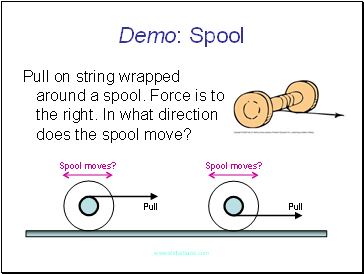Demo: Spool

Pull on string wrapped around a spool. Force is to the right. In what direction does the spool move?

Pull

Pull

Slide 38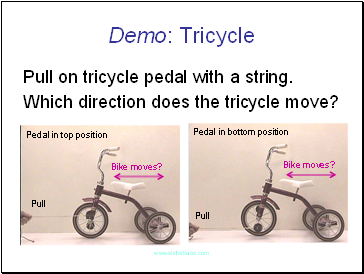Demo: Tricycle

Pull on tricycle pedal with a string.

Which direction does the tricycle move?

Pull

Pull

Pedal in top position

Pedal in bottom position

Slide 39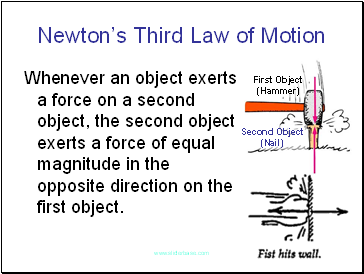## Newton’s Third Law of Motion

Whenever an object exerts a force on a second object, the second object exerts a force of equal magnitude in the opposite direction on the first object.

First Object

(Hammer)

Second Object

(Nail)

Slide 40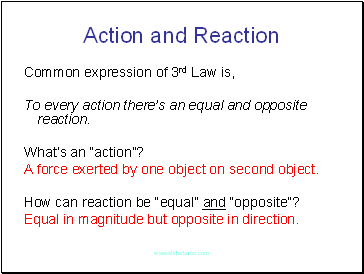## Action and Reaction

Common expression of 3rd Law is,

To every action there’s an equal and opposite reaction.

What’s an “action”?

A force exerted by one object on second object.

How can reaction be “equal” and “opposite”?

Equal in magnitude but opposite in direction.

Slide 41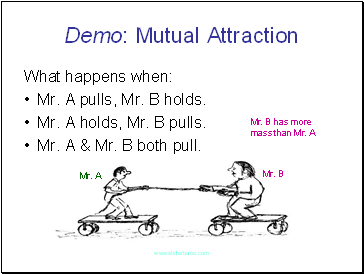Demo: Mutual Attraction

What happens when:

Mr. A pulls, Mr. B holds.

Mr. A holds, Mr. B pulls.

Mr. A & Mr. B both pull.

Mr. B

Mr. A

Mr. B has more mass than Mr. A

Slide 42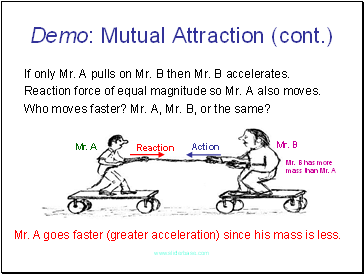Demo: Mutual Attraction (cont.)

If only Mr. A pulls on Mr. B then Mr. B accelerates.

Reaction force of equal magnitude so Mr. A also moves.

Who moves faster? Mr. A, Mr. B, or the same?

Action

Reaction

Mr. A

Mr. B

Mr. A goes faster (greater acceleration) since his mass is less.

Mr. B has more mass than Mr. A

Slide 43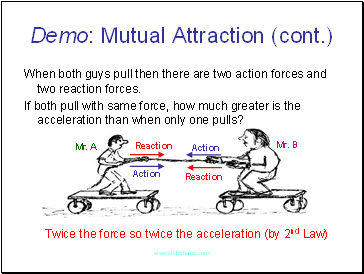Demo: Mutual Attraction (cont.)

When both guys pull then there are two action forces and two reaction forces.

If both pull with same force, how much greater is the acceleration than when only one pulls?

Action

Reaction

Mr. A

Mr. B

Twice the force so twice the acceleration (by 2nd Law)

Reaction

Action

Slide 44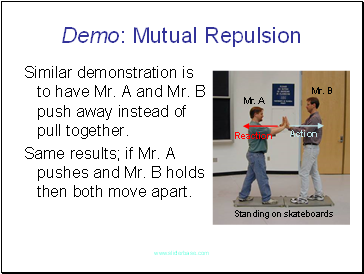Demo: Mutual Repulsion

Similar demonstration is to have Mr. A and Mr. B push away instead of pull together.

Same results; if Mr. A pushes and Mr. B holds then both move apart.

Standing on skateboards

Action

Reaction

Mr. A

Mr. B

Slide 45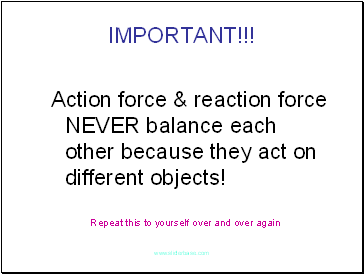Go to page:
1  2  3  4  5  6  7